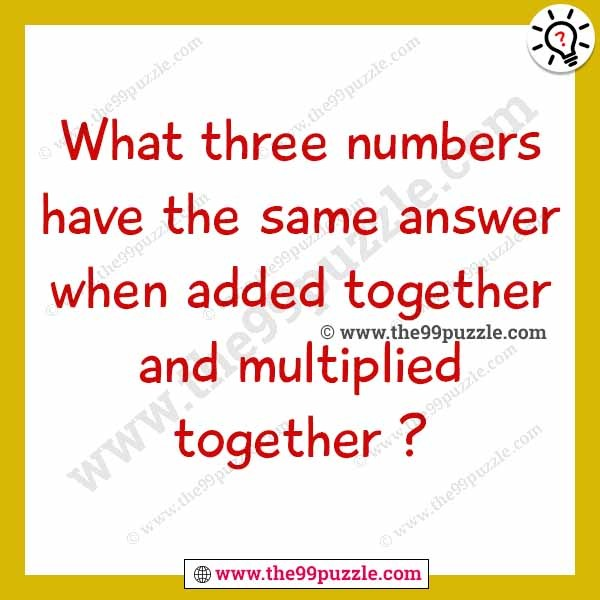# What three number have the same answer when added together and multiplied together – Puzz124

What three number have the same answer when added together and multiplied together?###### Explanation:

The three numbers are 1 2 3